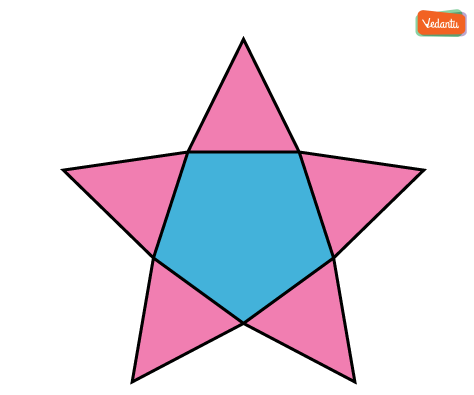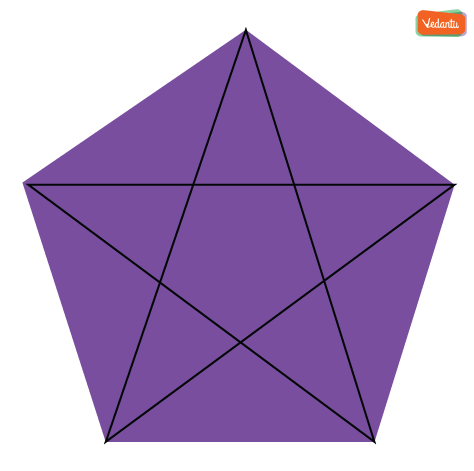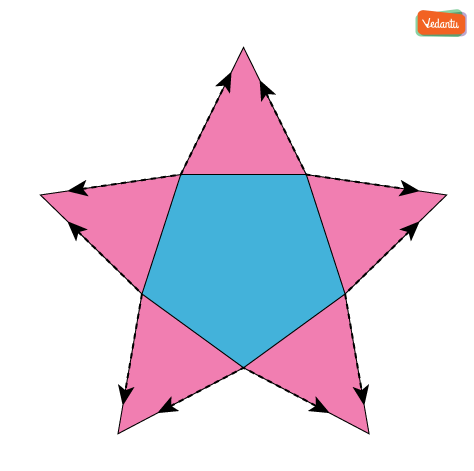Courses
Courses for Kids
Free study material
Offline Centres
More

# PentagramLast updated date: 22nd Nov 2023
Total views: 299.4k
Views today: 3.99k## Pentagram Meaning

In Euclidean geometry, Pentagram is a polygon-shaped like a five-pointed star. The five-pointed pentagram star has been popular in human history for many years. It is sometimes also known as a star –Pentagram because of its shape and the pentagon in its center, which also describes the origin of the word pentagram.

In the past, the symbol of pentagram has also been used as a holy sign that denoted goodness, and for protection against evil. Refer below to the pentagram star.### Use and Significance of Pentagram

The pentagram with a pentagon in the center has its own significance for ages. The shape is significant in many cultures. While some believe that its five edges denote a mystical pentagram sign that tells us about the 5 elements of nature i.e. air, water, fire, earth, and spirit which allows us to attain greater power, health, happiness, wisdom, and prosperity. Others say that the pentagram triangle denotes the five senses of hearing, sight, smell, touch, and taste.

### Construct a Regular Pentagram

There are various ways in which a regular pentagram can be constructed. However, let’s check for the two most common and interesting ones i.e.;

1. Inside a Regular Pentagon

You can construct a regular pentagram inside a regular pentagon by constructing its diagonals. To construct a pentagram inside a Pentagon, draw 2 diagonals each from all the five vertices of the pentagon. And look, your pentagram is ready!Now, let us check how to construct a pentagram outside a regular pentagon

1. Outside a Regular Pentagon

You can also construct a regular pentagram outside a regular pentagon by stretching out its five sides. In order to draw a Pentagram outside a pentagon, extend each side of the pentagon in a way that they bisect each other as shown in the image.

And guess what you make a pentagram again!### Golden Ratios in Pentagram

The pentagram consists of a unique number hidden inside. This special number is known as the Golden Ratio, which equals to about 1.618.

M/n = 1.618...

N/o = 1.618...

O/p = 1.618...

When you draw this, you will obtain the 4 lengths measuring as below:

m = 216,

n = 133,

o = 82,

p = 51.

Having said that, let's check to see what the ratios are:

216 ÷ 133 = 1.624...

133 ÷ 82 = 1.622...

82 ÷ 51 = 1.608...

Idea is to use Golden Ratio between m/n, n/o, and o/p which is equivalent to around 1.618.

Inner side length d is given so

o = 1.618 * p

n = 1.618 * o

m = 1.618 * n

MN, NO and OP are equals (both side of regular pentagram)

So MN = NO = OP = o and OP is given by p.

### The Pentacle

Do you know the pentacle's meaning? A pentagram is also known in the name of a pentacle which is typically a representative of a magical object or a talisman. This is disc-shaped and inscribed with a pentagram, also signifying the element of earth. The pentagram is also spoken about with reference to pentacle protection given its significance as a talisman object.

### Solved Examples

Example:

State whether the given statements are true or false with respect to a Pentagram:

1. A regular pentagon can be drawn inside a regular pentagram.

2. There exist a hexagon in the center of a regular pentagram

3. There is an octagon inside a pentagram.

4. The pentagram is popular as a magical symbol.

Solution:

1. True, a regular pentagon can be drawn inside a regular pentagram across its diagonals.

2. False, there is a regular pentagon on the interior of a regular pentagram.

3. False, there is no octagon inside a pentagon.

4. True, the pentagram is popular as a magical sign.

### Fun Facts

• The Pentagram is a 5-pointed star formed by drawing a continuous line in five straight segments.

• A pentagram can be drawn inside and outside a regular pentagon.

• There are five congruent isosceles triangles in a regular pentagram.

• There is a regular pentagon on the interior of a regular pentagram.

• You can easily construct a pentagram by stretching out the sides of a regular pentagon using a ruler.

• You can draw two diagonals from each vertex of a regular pentagon to create a pentagram.

## FAQs on Pentagram

Q1. What are the Different Types of Pentagrams?

Answer: Pentagrams are typically classified into regular pentagrams and irregular pentagrams.

1. Regular Pentagrams

A regular pentagram has the following properties:

• A regular pentagon in the center.

• Five congruent isosceles triangles.

1. Irregular Pentagrams

An irregular pentagram has the following properties:

• An irregular pentagon in its center.

• 5 triangles that are not congruent.

Q2. What are the Angles Formed in a Regular Pentagram?

Answer: We are already aware that there is a regular pentagon formed in the centre of a regular pentagram.

The angles formed in a pentagram are described as below:

1. Interior Angles

• Each interior angle of this regular pentagon equals to 108°.

• ∠MNO = ∠NOP = ∠OPQ = ∠PQM = ∠QMN = 108°.

2. Supplementary Angles

As mentioned above, the interior angles of the pentagon in the center measures 108°.

Thus, all the respective supplementary angles will be equal to 72°.

Now, since all the 5 triangles of the pentagram are isosceles, their base angles are congruent.

Therefore, all the ten base angles of the 5 triangles will measure 72°.

∠SMN = ∠SNM = ∠TNO = ∠TON = ∠UOP = ∠UPO = ∠VPQ = ∠VQP = ∠RQM = ∠RMQ = 72°.

3. Remaining Angles

Now, we are aware of all the angles of the pentagram, besides the ones which give it the shape of a star. Let's explore for more!

We know that the sum of the interior angles of a triangle is 180°.

Now, let's see the triangle SMN,

∠S = 180° − (72° + 72°) = 36°.

In the same manner, all the other angles of the remaining triangles of the pentagram will be calculated similarly.

∠T = ∠U = ∠V = ∠R = 36°.